This book is archived and will be removed July 6, 2022. Please use the updated version.

Temperature and Heat

# 3 Thermometers and Temperature Scales

### Learning Objectives

By the end of this section, you will be able to:

• Describe several different types of thermometers
• Convert temperatures between the Celsius, Fahrenheit, and Kelvin scales

Any physical property that depends consistently and reproducibly on temperature can be used as the basis of a thermometer. For example, volume increases with temperature for most substances. This property is the basis for the common alcohol thermometer and the original mercury thermometers. Other properties used to measure temperature include electrical resistance, color, and the emission of infrared radiation ((Figure)).

Because many physical properties depend on temperature, the variety of thermometers is remarkable. (a) In this common type of thermometer, the alcohol, containing a red dye, expands more rapidly than the glass encasing it. When the thermometer’s temperature increases, the liquid from the bulb is forced into the narrow tube, producing a large change in the length of the column for a small change in temperature. (b) Each of the six squares on this plastic (liquid crystal) thermometer contains a film of a different heat-sensitive liquid crystal material. Below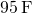, all six squares are black. When the plastic thermometer is exposed to a temperature of, the first liquid crystal square changes color. When the temperature reaches above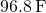, the second liquid crystal square also changes color, and so forth. (c) A firefighter uses a pyrometer to check the temperature of an aircraft carrier’s ventilation system. The pyrometer measures infrared radiation (whose emission varies with temperature) from the vent and quickly produces a temperature readout. Infrared thermometers are also frequently used to measure body temperature by gently placing them in the ear canal. Such thermometers are more accurate than the alcohol thermometers placed under the tongue or in the armpit. (credit b: modification of work by Tess Watson; credit c: modification of work by Lamel J. Hinton, U.S. Navy)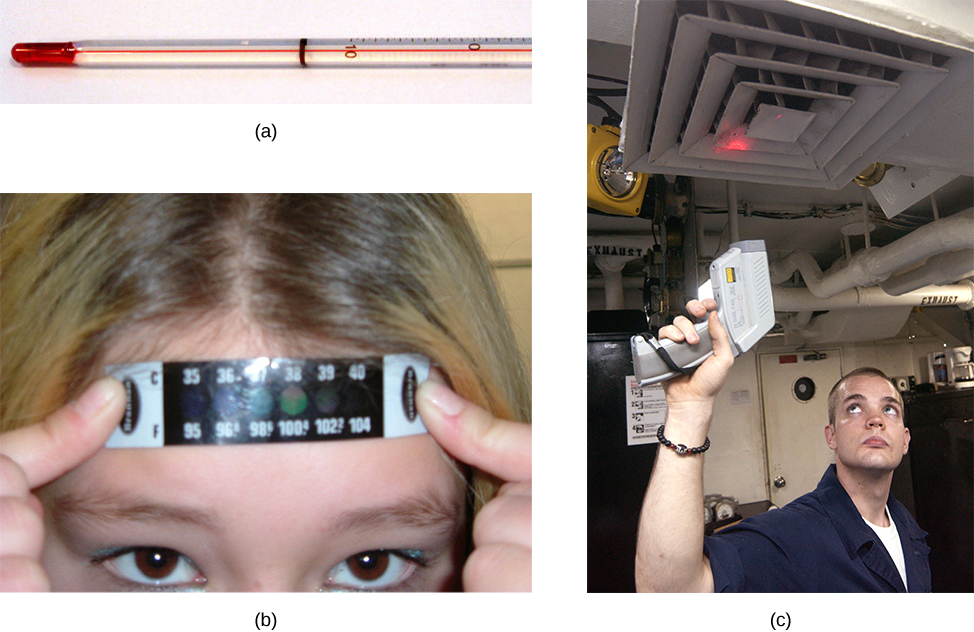Thermometers measure temperature according to well-defined scales of measurement. The three most common temperature scales are Fahrenheit, Celsius, and Kelvin. Temperature scales are created by identifying two reproducible temperatures. The freezing and boiling temperatures of water at standard atmospheric pressure are commonly used.

On the Celsius scale, the freezing point of water is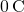and the boiling point is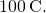The unit of temperature on this scale is the degree Celsius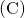. The Fahrenheit scale (still the most frequently used for common purposes in the United States) has the freezing point of water at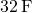and the boiling point at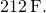Its unit is the degree Fahrenheit (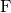). You can see that 100 Celsius degrees span the same range as 180 Fahrenheit degrees. Thus, a temperature difference of one degree on the Celsius scale is 1.8 times as large as a difference of one degree on the Fahrenheit scale, or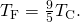The definition of temperature in terms of molecular motion suggests that there should be a lowest possible temperature, where the average kinetic energy of molecules is zero (or the minimum allowed by quantum mechanics). Experiments confirm the existence of such a temperature, called absolute zero. An absolute temperature scale is one whose zero point is absolute zero. Such scales are convenient in science because several physical quantities, such as the volume of an ideal gas, are directly related to absolute temperature.

The Kelvin scale is the absolute temperature scale that is commonly used in science. The SI temperature unit is the kelvin, which is abbreviated K (not accompanied by a degree sign). Thus 0 K is absolute zero. The freezing and boiling points of water are 273.15 K and 373.15 K, respectively. Therefore, temperature differences are the same in units of kelvins and degrees Celsius, or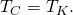The relationships between the three common temperature scales are shown in (Figure). Temperatures on these scales can be converted using the equations in (Figure).

Relationships between the Fahrenheit, Celsius, and Kelvin temperature scales are shown. The relative sizes of the scales are also shown.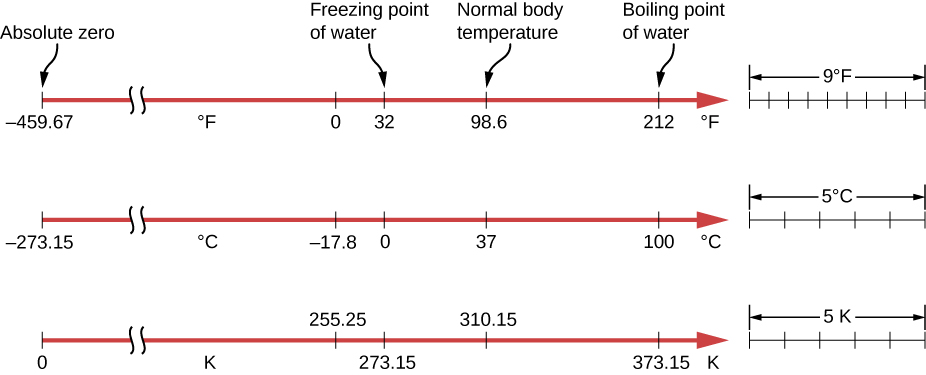Temperature Conversions
To convert from… Use this equation…
Celsius to Fahrenheit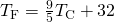Fahrenheit to Celsius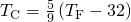Celsius to Kelvin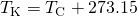Kelvin to Celsius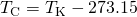Fahrenheit to Kelvin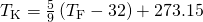Kelvin to Fahrenheit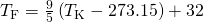To convert between Fahrenheit and Kelvin, convert to Celsius as an intermediate step.

Converting between Temperature Scales: Room Temperature “Room temperature” is generally defined in physics to be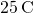. (a) What is room temperature in? (b) What is it in K?

Strategy To answer these questions, all we need to do is choose the correct conversion equations and substitute the known values.

Solution To convert from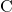to, use the equation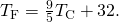Substitute the known value into the equation and solve: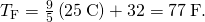Similarly, we find that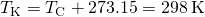.

The Kelvin scale is part of the SI system of units, so its actual definition is more complicated than the one given above. First, it is not defined in terms of the freezing and boiling points of water, but in terms of the triple point. The triple point is the unique combination of temperature and pressure at which ice, liquid water, and water vapor can coexist stably. As will be discussed in the section on phase changes, the coexistence is achieved by lowering the pressure and consequently the boiling point to reach the freezing point. The triple-point temperature is defined as 273.16 K. This definition has the advantage that although the freezing temperature and boiling temperature of water depend on pressure, there is only one triple-point temperature.

Second, even with two points on the scale defined, different thermometers give somewhat different results for other temperatures. Therefore, a standard thermometer is required. Metrologists (experts in the science of measurement) have chosen the constant-volume gas thermometer for this purpose. A vessel of constant volume filled with gas is subjected to temperature changes, and the measured temperature is proportional to the change in pressure. Using “TP” to represent the triple point,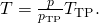The results depend somewhat on the choice of gas, but the less dense the gas in the bulb, the better the results for different gases agree. If the results are extrapolated to zero density, the results agree quite well, with zero pressure corresponding to a temperature of absolute zero.

Constant-volume gas thermometers are big and come to equilibrium slowly, so they are used mostly as standards to calibrate other thermometers.

### Summary

• Three types of thermometers are alcohol, liquid crystal, and infrared radiation (pyrometer).
• The three main temperature scales are Celsius, Fahrenheit, and Kelvin. Temperatures can be converted from one scale to another using temperature conversion equations.
• The three phases of water (ice, liquid water, and water vapor) can coexist at a single pressure and temperature known as the triple point.

### Conceptual Questions

If a thermometer is allowed to come to equilibrium with the air, and a glass of water is not in equilibrium with the air, what will happen to the thermometer reading when it is placed in the water?

Give an example of a physical property that varies with temperature and describe how it is used to measure temperature.

### Problems

While traveling outside the United States, you feel sick. A companion gets you a thermometer, which says your temperature is 39. What scale is that on? What is your Fahrenheit temperature? Should you seek medical help?

That must be Celsius. Your Fahrenheit temperature is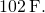Yes, it is time to get treatment.

What are the following temperatures on the Kelvin scale?

(a)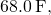an indoor temperature sometimes recommended for energy conservation in winter

(b)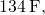one of the highest atmospheric temperatures ever recorded on Earth (Death Valley, California, 1913)

(c)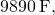the temperature of the surface of the Sun

(a) Suppose a cold front blows into your locale and drops the temperature by 40.0 Fahrenheit degrees. How many degrees Celsius does the temperature decrease when it decreases by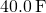? (b) Show that any change in temperature in Fahrenheit degrees is nine-fifths the change in Celsius degrees

a.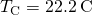; b. We know that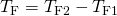. We also know that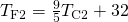and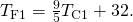So, substituting, we have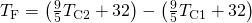. Partially solving and rearranging the equation, we have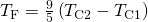. Therefore,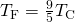.

An Associated Press article on climate change said, “Some of the ice shelf’s disappearance was probably during times when the planet was 36 degrees Fahrenheit (2 degrees Celsius) to 37 degrees Fahrenheit (3 degrees Celsius) warmer than it is today.” What mistake did the reporter make?

(a) At what temperature do the Fahrenheit and Celsius scales have the same numerical value? (b) At what temperature do the Fahrenheit and Kelvin scales have the same numerical value?

a.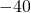; b. 575 K

A person taking a reading of the temperature in a freezer in Celsius makes two mistakes: first omitting the negative sign and then thinking the temperature is Fahrenheit. That is, the person reads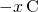as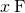. Oddly enough, the result is the correct Fahrenheit temperature. What is the original Celsius reading? Round your answer to three significant figures.

### Glossary

absolute temperature scale
scale, such as Kelvin, with a zero point that is absolute zero
absolute zero
temperature at which the average kinetic energy of molecules is zero
Celsius scale
temperature scale in which the freezing point of water isand the boiling point of water is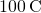degree Celsius
() unit on the Celsius temperature scale
degree Fahrenheit
() unit on the Fahrenheit temperature scale
Fahrenheit scale
temperature scale in which the freezing point of water isand the boiling point of water is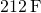Kelvin scale (K)
temperature scale in which 0 K is the lowest possible temperature, representing absolute zero
triple point
pressure and temperature at which a substance exists in equilibrium as a solid, liquid, and gas